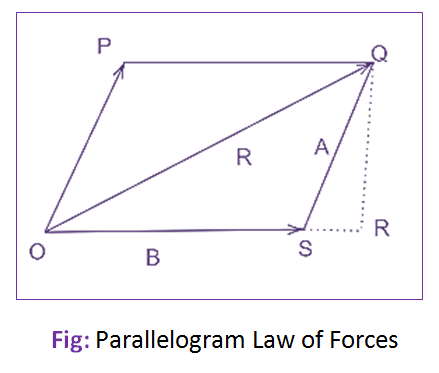# State and Explain Parallelogram Law of Forces

## Parallelogram Law of Forces:

It states that “If two forces are acting simultaneously on a particle, be represented in magnitude and direction by the two adjacent sides of a parallelogram, their results may be represented in magnitude and direction by the diagonal of the parallelogram which passes through their point of intersection“.Mathematically, the resultant force,

Where, F1 and F2 = Forces whose resultant is required to be found out.
θ = Angle between the forces F1 and F2
α = Angle which the resultant force makes with one of the forces say F1.